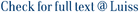In this paper, a Banach space framework is introduced in order to deal with finite-dimensional path-dependent stochastic differential equations. A version of Kolmogorov backward equation is formulated and solved both in the space of Lp paths and in the space of continuous paths using the associated stochastic differential equation, thus establishing a relation between path-dependent SDEs and PDEs in analogy with the classical case. Finally, it is shown how to establish a connection between such Kolmogorov equation and the analogue finite-dimensional equation that can be formulated in terms of the path-dependent derivatives recently introduced by Dupire, Cont and Fournié.

An infinite-dimensional approach to path-dependent Kolmogorov equations / Flandoli, Franco; Zanco, Giovanni Alessandro. - In: ANNALS OF PROBABILITY. - ISSN 0091-1798. - 44:4(2016), pp. 2643-2693. [10.1214/15-AOP1031]

### An infinite-dimensional approach to path-dependent Kolmogorov equations

#### Abstract

In this paper, a Banach space framework is introduced in order to deal with finite-dimensional path-dependent stochastic differential equations. A version of Kolmogorov backward equation is formulated and solved both in the space of Lp paths and in the space of continuous paths using the associated stochastic differential equation, thus establishing a relation between path-dependent SDEs and PDEs in analogy with the classical case. Finally, it is shown how to establish a connection between such Kolmogorov equation and the analogue finite-dimensional equation that can be formulated in terms of the path-dependent derivatives recently introduced by Dupire, Cont and Fournié.
##### Scheda breve Scheda completa Scheda completa (DC)2016
Path-dependent SDEs, path-dependent PDEs, delay equations, stochastic calculus in Banach spaces, Kolmogorov equations
An infinite-dimensional approach to path-dependent Kolmogorov equations / Flandoli, Franco; Zanco, Giovanni Alessandro. - In: ANNALS OF PROBABILITY. - ISSN 0091-1798. - 44:4(2016), pp. 2643-2693. [10.1214/15-AOP1031]
File in questo prodotto:
File
AOP1031 (1).pdf

Open Access

Tipologia: Versione dell'editore
Licenza: DRM (Digital rights management) non definiti
Dimensione 391.11 kB
Utilizza questo identificativo per citare o creare un link a questo documento: `https://hdl.handle.net/11385/183481`
•30
•23